Author: Oscar Cronquist Article last updated on May 04, 2022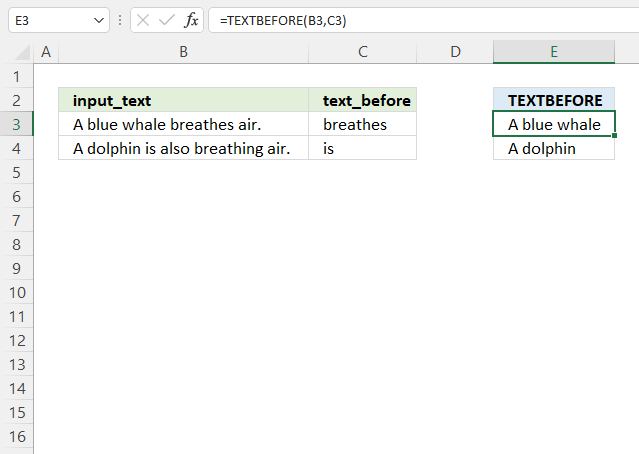The TEXTBEFORE function extracts a string before a specific substring from a given value.

Formula in cell E3:

=TEXTBEFORE(B3, C3)

The TEXTBEFORE function is available to Excel 365 users.

## 1. TEXTBEFORE Function Syntax

TEXTBEFORE(input_text,text_before, [n], [ignore_case])

## 2. TEXTBEFORE Function Arguments

 input_text Required. The original string. text_before Required. The string to search for, text before this string is extracted. [n] Optional.  The instance of text_before string, default is 1. [ignore_case] Optional. FALSE represents case sensitive search, default is TRUE.

## 3. TEXTBEFORE Function example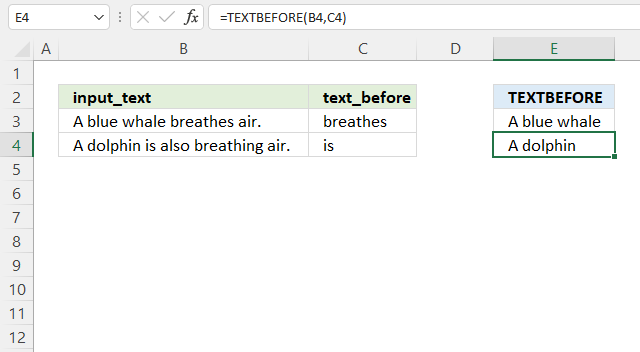Formula in cell E4:

=TEXTBEFORE(B4, C4)

### Explaining formula

#### Step 1 - TEXTBEFORE function

TEXTBEFORE(input_text,text_before, [n], [ignore_case])

#### Step 2 - Populate arguments

input_text - B4
text_before - C4
[n] - Optional, default is 1.
[ignore_case] - Optional, default is TRUE meaning not case sensitive

#### Step 3 - Evaluate function

TEXTBEFORE(B4, C4)

becomes

TEXTBEFORE("A dolphin is also breathing air.", "is")

and returns "A dolphin".

## 4. TEXTBEFORE Function - case sensitive example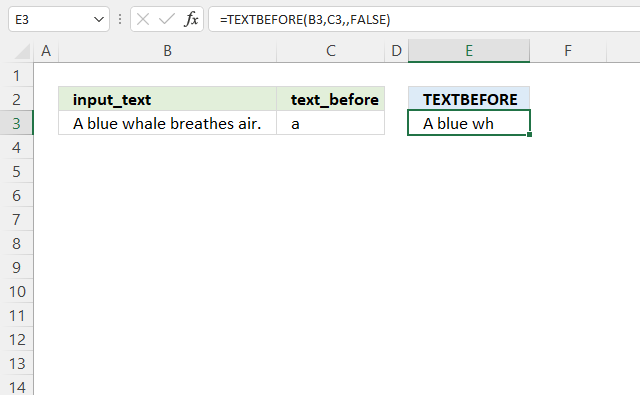This example shows how to use the TEXTBEFORE function also considering upper and lower letters.

Formula in cell E4:

=TEXTBEFORE(B4, C4,,FALSE)

### Explaining formula

#### Step 1 - TEXTBEFORE function

TEXTBEFORE(input_text,text_before, [n], [ignore_case])

#### Step 2 - Populate arguments

input_text - B4
text_before - C4
[n] - Optional, default is 1.
[ignore_case] - FALSE meaning case sensitive.

#### Step 3 - Evaluate function

TEXTBEFORE(B4, C4,,FALSE)

becomes

TEXTBEFORE("A blue whale breathes air.", "a", , FALSE)

and returns "A blue wh".

## 5. TEXTBEFORE Function alternative (earlier Excel versions)

The TEXTBEFORE function lets you perform both a regular and a case-sensitive search based on the fourth argument [ignore_case]. The SEARCH and FIND  functions are used in the alternative formulas below.

## 5.1 TEXTBEFORE Function alternative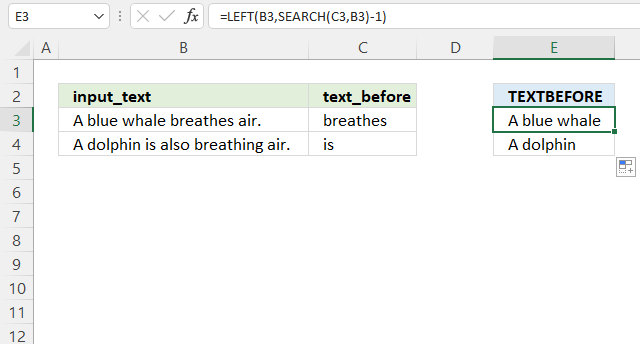This formula works in all Excel versions.

Formula in cell E4:

=LEFT(B3, SEARCH(C3, B3)-1)

### Explaining formula

#### Step 1 - Find character position of substring

The SEARCH function returns a number representing the position of character at which a specific text string is found reading left to right. It is NOT a case-sensitive search.

SEARCH(find_text,within_text, [start_num])

SEARCH(C3, B3)-1

becomes

SEARCH("breathes", "A blue whale breathes air.")-1

becomes

14-1 equals 13.

#### Step 2 - Extract text before based on position

The LEFT function extracts a specific number of characters always starting from the left.

LEFT(text, [num_chars])

LEFT(B3, SEARCH(C3, B3)-1)

becomes

LEFT("A blue whale breathes air.", 13)

and returns "A blue whale ".

## 5.2 TEXTBEFORE Function alternative - case sensitive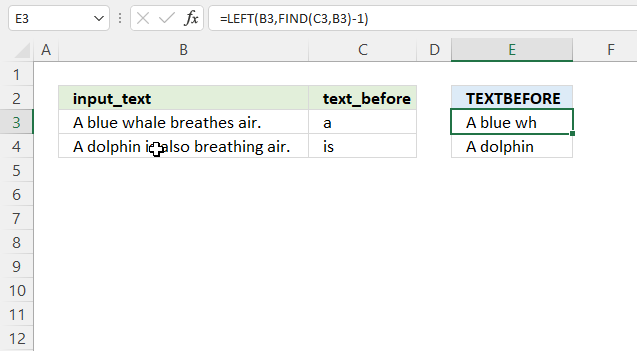This formula performs a case-sensitive search in order to extract text before the given substring.

Formula in cell E3:

=LEFT(B3, FIND(C3, B3)-1)

### Explaining formula

#### Step 1 - Find character position of substring

The FIND function returns a number representing the position of character at which a specific text string is found reading left to right. This function performs a case-sensitive search.

FIND(find_text,within_text, [start_num])

FIND(C3, B3)-1

becomes

FIND("breathes", "A blue whale breathes air.")-1

becomes

10-1 equals 9.

#### Step 2 - Extract text before based on position

The LEFT function extracts a specific number of characters always starting from the left.

LEFT(text, [num_chars])

LEFT(B3, FIND(C3, B3)-1)

becomes

LEFT(B3, 9)

becomes

LEFT("A blue whale breathes air.", 9)

and returns "A blue wh".